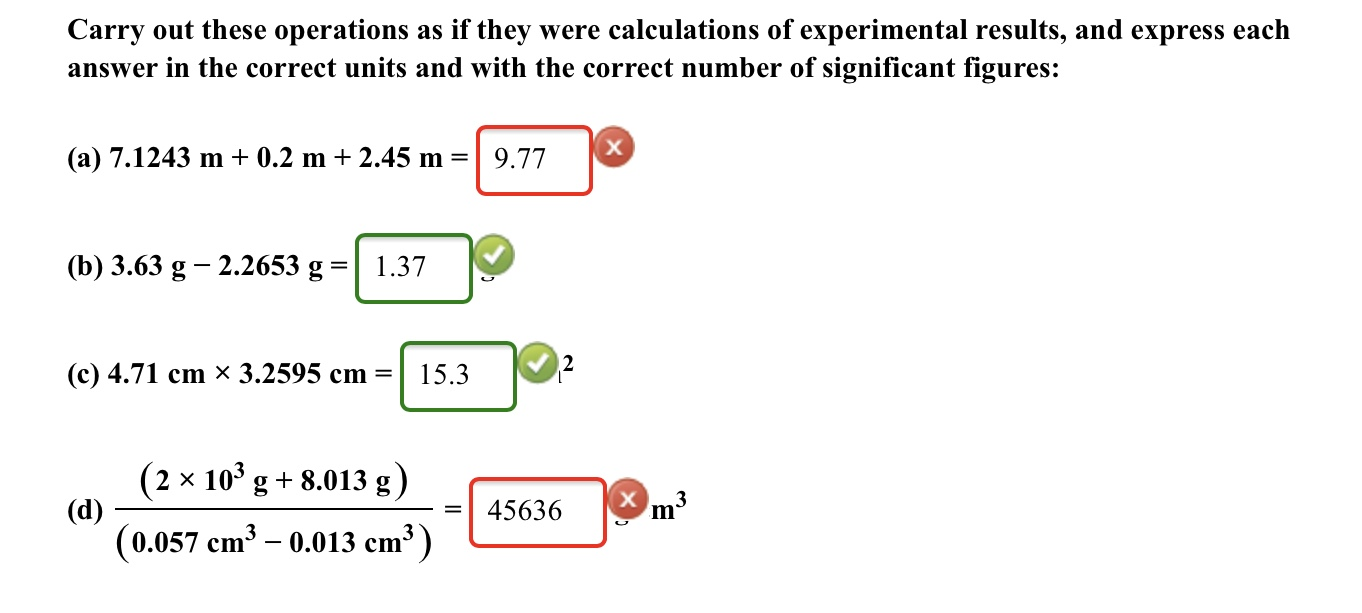# Carry out these operations as if they were calculations of experimental results, and express each answer in the correct units and with the correct number of significant figures: (a) 7.1243 m + 0.2 m + 2.45 m = (b) 3.63 g- 2.2653 g = (c) 4.71 cm x 3.2595 cm = (d) (2x10^3 g + 8.013 g)/(0.057 cm^3 - 0.013 cm^3) =